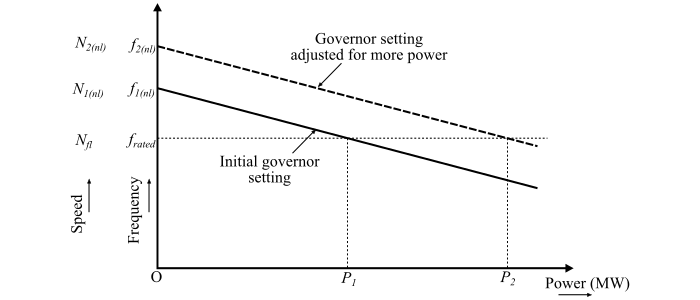# Prime-Mover Governor Characteristics (Synchronous Generator)

The prime-mover governor characteristic is a graph plotted between the speed of the prime-mover (or generator frequency) and the active power. A typical prime-mover governor characteristic is shown in the figure.The prime mover characteristic usually drawn as a straight line, but the actual characteristic has a slight curve.

For the successful parallel operation of the alternators or synchronous generators, the load-speed characteristics of the prime movers should be drooping, i.e., the speed of the prime mover should decrease slightly with increasing loads. The drooping characteristic provides inherent stability of the operation of an alternator when paralleled with the other alternators. The alternators with zero droop are known as isochronous alternators and are inherently unstable when operated in parallel. These alternators are subjected to unexpected load swings, unless controlled by the solid-state regulators.

The speed droop is also known as generator droop or inherent speed regulation or governor speed regulation (GSR). The speed droop is usually expressed as a percentage of the full-load speed as follows.

$$\mathrm{Speed\:droop =\frac{𝑁_{𝑛𝑙} − 𝑁_{𝑓𝑙}}{𝑁_{𝑓𝑙}} \times 100\% =\frac{𝑓_{𝑛𝑙} − 𝑓_{𝑟𝑎𝑡𝑒𝑑}}{𝑓_{𝑟𝑎𝑡𝑒𝑑}} \times 100\%}$$

Where,

• $𝑁_{𝑓𝑙}$ is full-load speed.
• $𝑁_{𝑛𝑙}$ is no-load speed.
• $𝑓_{𝑛𝑙}$ is no-load frequency in Hz.
• $𝑓_{𝑟𝑎𝑡𝑒𝑑}$ is the rated frequency in Hz.

In practice, the speed droop normally varies from 2% to 4% from no-load to full-load.

The amount of power generated by an alternator is determined by its prime mover. The speed of the prime mover is fixed while its torque can be varied. This is done by adjusting the tension of the spring of the speed governor mechanism. The above figure shows the effect of variation of the governor characteristics. Here, the load-speed characteristic is shifted to a new position parallel to the initial position.

Now, when two alternators are operating in parallel, then,

Case 1 – An increase in governor set points on one of them,

• Increases the system frequency, and

• Increases the power delivered by that alternator and decreases the power delivered by the other alternator.

Case 2 – The field current of the second alternator is increased,

• Increases the terminal voltage of the system, and

• Increases the reactive power (Q) delivered by that alternator, while reduces the reactive power delivered by the other alternator.